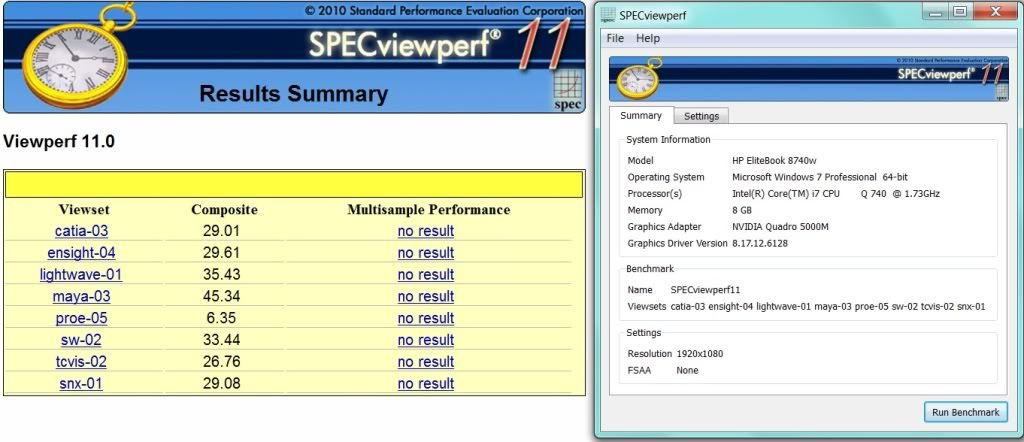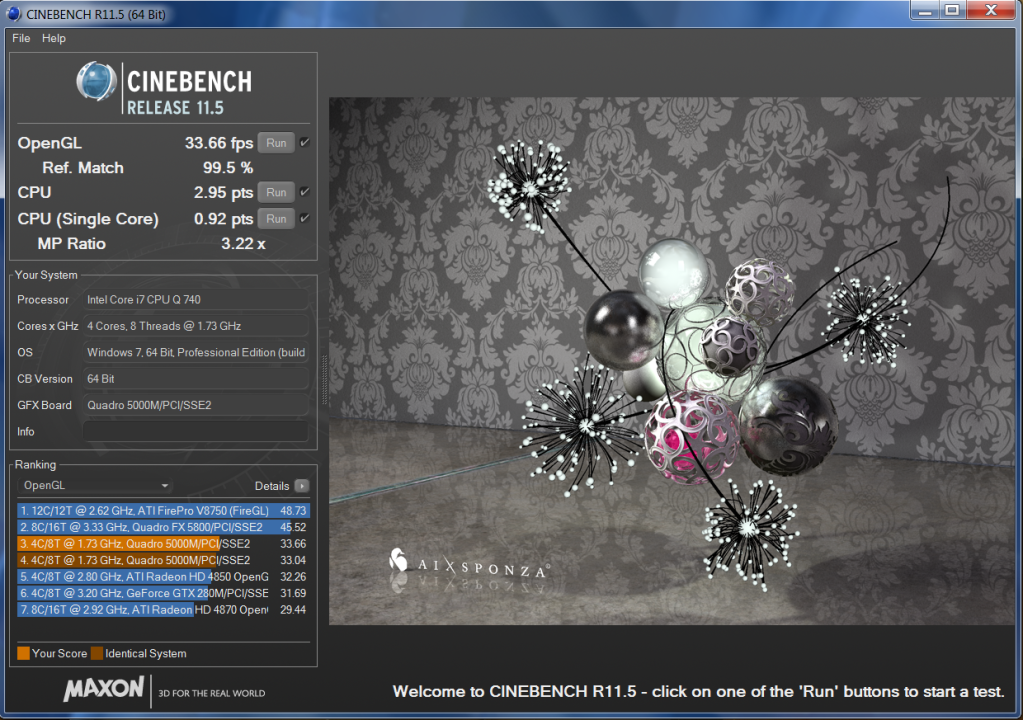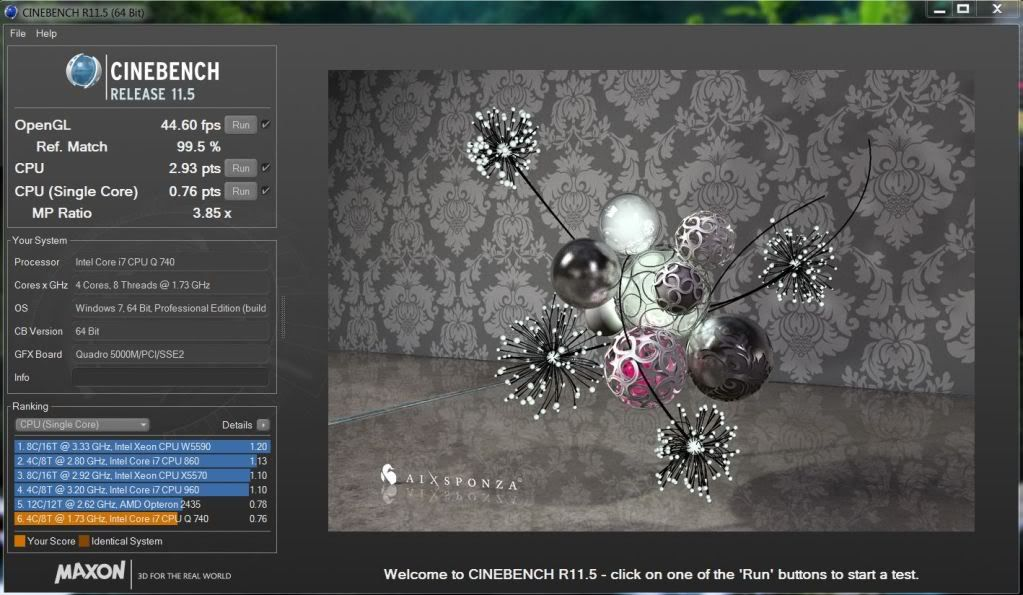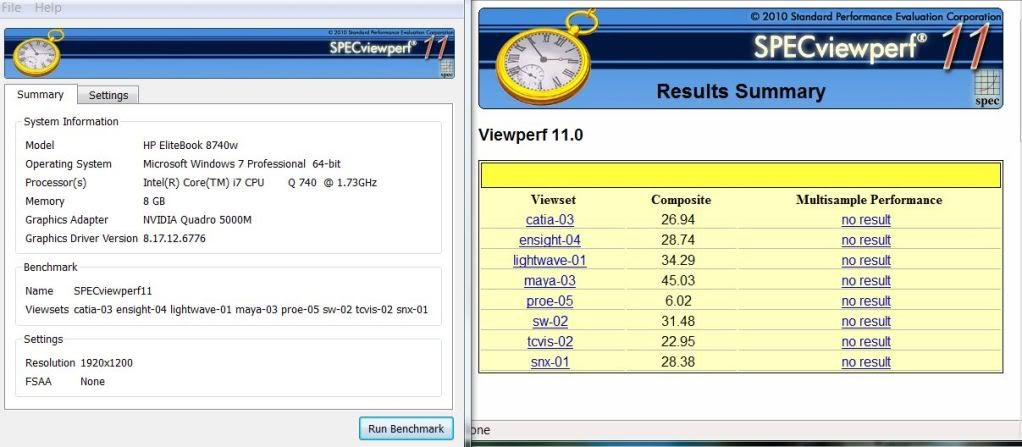Discussion in 'HP Business Class Notebooks' started by Siorah, Nov 14, 2010.

Not open for further replies.
1. ### SiorahBeware of Squirrels!

Reputations:
607
Messages:
734
8
Trophy Points:
31
updated post to reflect that jud. some people like me need stable fast memory, if i lost that video editing would be seriously slow work! lol

2. ### knight427theenemysgateisdown

Reputations:
1,158
Messages:
1,616
127
Trophy Points:
81
I ran SPECviewperf on my replacement laptop (after updating drivers)

I accidentally ran it on 1080p first.I shut it down for 30min to cool down and ran it again on 1200p.Here I installed the drivers from nVIDIA's website...1200p.3. ### MaruNotebook Consultant

Reputations:
136
Messages:
235
88
Trophy Points:
41
Looks like driver 8.17.12.6128 on 5000M provides a little speedup
for Catia (~13%) and Solidworks (~9%) over driver 8.17.12.5947.

Nice to see a comparison of WUXGA (1920x1200) and FHD (1920x1080) numbers.
WUXGA displays ~11% more pixels in ~0% to ~2.7% more time per frame over FHD.

Driver 8.17.12.6658 does not make much difference over 8.17.12.6128
(maybe ~4% worse for Teamcenter Vis).

Code:
```<pre style='font-size: xx-small; font-family: 'Lucida Console', monospace'>
740QM 8GB DC2 WUXGA

catia-03 (CATIA V5R19,V6R2009)
5000M 12.5947 1200p ))))))))))))))))))))))))) 25.10
5000M 12.6128 1200p ))))))))))))))))))))))))))))) 28.57
5000M 12.6128 1080p ))))))))))))))))))))))))))))) 29.01
5000M 12.6658 1200p ))))))))))))))))))))))))))))) 29.10
ensight-04 (EnSight 8.2)
5000M 12.5947 1200p ))))))))))))))))))))))))))))) 28.63
5000M 12.6128 1200p ))))))))))))))))))))))))))))) 28.84
5000M 12.6128 1080p )))))))))))))))))))))))))))))) 29.61
5000M 12.6658 1200p ))))))))))))))))))))))))))))) 28.92
lightwave-01 (LightWave 9.6)
5000M 12.5947 1200p ))))))))))))))))))))))))))))))))))) 34.69
5000M 12.6128 1200p ))))))))))))))))))))))))))))))))))) 35.49
5000M 12.6128 1080p ))))))))))))))))))))))))))))))))))) 35.43
5000M 12.6658 1200p ))))))))))))))))))))))))))))))))))) 35.43
maya-03 (Maya 2009)
5000M 12.5947 1200p )))))))))))))))))))))))))))))))))))))))))))) 43.89
5000M 12.6128 1200p ))))))))))))))))))))))))))))))))))))))))))))) 45.34
5000M 12.6128 1080p ))))))))))))))))))))))))))))))))))))))))))))) 45.52
5000M 12.6658 1200p ))))))))))))))))))))))))))))))))))))))))))))) 45.48
proe-05 (Pro/Engineer Wildfire 5.0)
5000M 12.5947 1200p ))))))) 6.71
5000M 12.6128 1200p ))))))) 6.56
5000M 12.6128 1080p )))))) 6.35
5000M 12.6658 1200p ))))))) 6.58
sw-02 (Solidworks 2009 sp2)
5000M 12.5947 1200p )))))))))))))))))))))))))))))) 29.99
5000M 12.6128 1200p ))))))))))))))))))))))))))))))))) 32.84
5000M 12.6128 1080p ))))))))))))))))))))))))))))))))) 33.44
5000M 12.6658 1200p ))))))))))))))))))))))))))))))))) 32.95
tcvis-02 (Teamcenter Visualization Mockup)
5000M 12.5947 1200p ))))))))))))))))))))))))))) 26.82
5000M 12.6128 1200p )))))))))))))))))))))))))) 26.26
5000M 12.6128 1080p ))))))))))))))))))))))))))) 26.76
5000M 12.6658 1200p ))))))))))))))))))))))))) 25.04
snx-01 (Seimens NX 7)
5000M 12.5947 1200p )))))))))))))))))))))))))))) 28.13
5000M 12.6128 1200p ))))))))))))))))))))))))))))) 28.65
5000M 12.6128 1080p ))))))))))))))))))))))))))))) 29.08
5000M 12.6658 1200p ))))))))))))))))))))))))))))) 28.71
</pre>```

Last edited by a moderator: May 6, 2015
4. ### knight427theenemysgateisdown

Reputations:
1,158
Messages:
1,616
127
Trophy Points:
81
Cinnebench ran below. Also did 3DMark06 (link in sig).5. ### SiorahBeware of Squirrels!

Reputations:
607
Messages:
734
8
Trophy Points:
31
Bringing this back up to the front page again. As it's too important to sit back in page 4

6. ### SiorahBeware of Squirrels!

Reputations:
607
Messages:
734
8
Trophy Points:
31
Posting my Vbios Tutorial in this thread so it's easier to find for people, rather than it being buried in the history of the main thread!

============
Update History:
============

V.4 Changed tutorial to reflect F.0B Bios
V.3 Changed tutorial to reflect F.0A Bios
V.2 Changed tutorial to reflect F.09 Bios
V.1 Original Version

===========

VBIOS TUTORIAL

If you have a FirePro - this doesnt apply

Tutorial for updating Video Bios for Nvidia Cards

1) download the bios version F.0B and run it. Select USB KEY

2) when the hp usb tool runs just close it - don't let it install the freedos sys files because they don't work on the laptop

3) download the win98boot files from here Win98 Boot Files and follow the rest of that tutorial. the HPUSBFW is in c:\swsetup\sp52278\ run it with right click 'run as administrator'

4) OPEN the newly formatted drive. In another window, you will then need to go to c:\swsetup\sp52278\rompaq5) Unhide files in the folder window. Config.sys/Kernel.sys may be hidden if you can't see it, go to organise -> folder and search options, then the view tab. Click the button that says 'show hidden files, folders or drives'.If you can't see config.sys uncheck the second box, (hide protected operating system files) remember to change it back afterwards.if you are running F.08 or Above go to step 7

6) YOU NEED TO UPDATE THE BIOS TO AT LEAST F.08 FIRST BEFORE RUNNING THE VBIOS UPDATE.

for this you need to copy everything over that is in the ROMPAQ folder to the pendrive, then reboot to the usb drive by pressing F9 on the HP logo screen

press enter when you have the program open, and follow the on screen instructions.

let the computer power off and give it a few seconds (5-10 is ok) before restarting. then go back to Step 6.

7) IF YOU HAVE BIOS F.08 OR HIGHER ALREADY - you need to copy everything over from the ROMPAQ folder EXCEPT CONFIG.SYS that is in the folder to the pendrive, then reboot to the usb drive by pressing F9 on the HP logo screen

8) Now to update the Video Bios.

if you have an Nvidia 2800M (like i do)

type 'n 1.rom' (without the quotes) it will give you the current bios version with the new one. type 'y' and it will update. Skip to step 9

if you have an Nvidia 3800M

type 'n 2.rom' (without the quotes) it will give you the current bios version with the new one. type 'y' and it will update. Skip to step 9

if you have an Nvidia 5000M

type 'n 5.rom' (without the quotes) it will give you the current bios version with the new one. type 'y' and it will update. Skip to step 9

9) now press ctrl +alt + delete and let windows load. when it has done, choose shut down.

10) Turn on the pc again, and you will have a shiny new Nvidia vbios

======
UPDATE
======

This VBios update will enable you to:

> Use the CUDA acceleration in Adobe CS5

> Use CUDA for AVC-HD/PRO, VC-1, WMV-HD, CoreAVC if you don't know what these are, they are used in blu-ray playback.

> Run the IRAY Renderer in 3DS Max, GPU Rendering in Maya/Max

> Will fix the PCI-Express Timeout bug common with the 2800/3800M when running DirectX based overlay software (AutoCAD/Solidworks is an example)

> Will enable PCI-E Power saving on the cards (switching from PCI-E 2.0 to 1.1 when its IDLE)

> WILL ENABLE USE OF DRIVERS HIGHER THAN 250.13, AND THE DRIVERS FROM NVIDIA WHICH PROPERLY INSTALL PHYSX/OPEN CL/MAXIMISE/AUTOCAD SPEEDUP DRIVER. This is in caps, because it seems HP are still shipping the old Vbios' and people are having issues.

> Will enable users to use the Verde Drivers. If you have a 2800M, and wish to use the Nvidia Verde Driver, when you come to find the driver, select Quadro FX (notebook series) then 3800M on the driver page. The 2800M Driver is included in that package.

Also if you have a 2800M you can use the Quadro Embedded Driver. This is a different Branch than the Verde Driver, and is specifically for the 2800M you need to select Quadro Blade/Embedded Server, then 2800M

> If you are running any game made before 2008 - MAKE SURE, you run it in XP SERVICE PACK 2 mode in windows 7. This will stop a sporadic shutdown/BSOD

> It will stop the lag timeouts on the PCI-E bus, which sometimes result in a random STOP 0x124/0x116/0x125 error.

Note:

OLD 2800M Vbios Value: 62.92.B6.00.08/62.92.B8.00.02 NEW: 62.92.C0.00.03

OLD 3800M Vbios Value: 62.92.B9.00.02/62.92.BC.00.09 NEW: 62.92.C0.00.04

OLD 5000M Vbios Value: 70.00.35.00.06 NEW: 70.00.37.00.0A

(The 5000M No longer is shipped with this old Vbios, but check if you have one)

----------------------------

CURRENT NVidia Verde Driver - 2800M/3800M/5000M: 285.62 <- ok driver, USE THIS FOR AUTODESK 2012 SOFTWARE!!! (Max/Maya/Composite/Softimage/Mudbox etc) Fixed Directcompute apps, and opengl rendering

CURRENT BETA NVidia Verde Driver - 2800M/3800M/5000M: 290.36 <--- Some speedups on 3800M machines, best for 5000M at this time, stops 0x116/0x124 on some directx 9 applications

CURRENT NVidia Quadro Driver - 2800M Only: 276.28 <- Regressed to the older driver, not really good for us now.

CURRENT BETA NVidia Quadro Driver - 2800M Only: 285.58 <- Not really recommended, as they've broken something.

CURRENT HP Quadro Driver - 2800/3800M/5000M : 276.00 (8.17.12.7600) <- Fixes 0x116 SOMETIMES - Still not recommended for users with multi-display without a docking station (No AUDIO OUT ON DP+ -> HDMI) - Stop 0x124 Errors frequent on 3800M - STILL doesn't install OpenCL/CUDA/PhysX Properly.... sigh

7. ### SiorahBeware of Squirrels!

Reputations:
607
Messages:
734
8
Trophy Points:
31
New Nvidia 267.66 (2800M Only)/267.76 (2800/3800/5000)

The new drivers are very stable, with the Verde Tree (267.76) having game speedups and fixes.

The memory readout for nvidia control panel is fixed, as well as the readout now states what DirectX your GPU supports.

On the Quadro (267.66) line there are many fixes for software, mainly autoCad and softimage/pro engineer.

Not had issues yet on my system

but those of you on original factory installs, (i.e. You haven't reinstalled windows!) be careful of updates, as something installed really is breaking the newer drivers.

8. ### knight427theenemysgateisdown

Reputations:
1,158
Messages:
1,616
127
Trophy Points:
81
I installed 267.76 and ran the benchmarks. Here they are.

Big jump in fps.Mostly down a bit from last nVIDIA driver.3DMark06 - 13,148 :slightly less than HP drivers

3DMark11 - P2,114 :slightly better than both current HP and last nVIDIA

I'll be going back HP drivers soon so MDA works again.

9. ### naacostaNewbie

Reputations:
0
Messages:
6
0
Trophy Points:
5
i have flashed my bios to the f.OB version. i wanted to know how to update the video bios from here. the tutorial stoped at the f.OA version.

Reputations:
607
Messages:
734